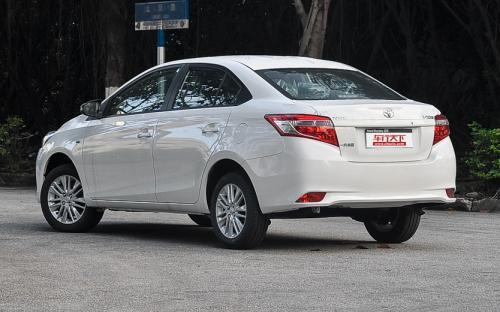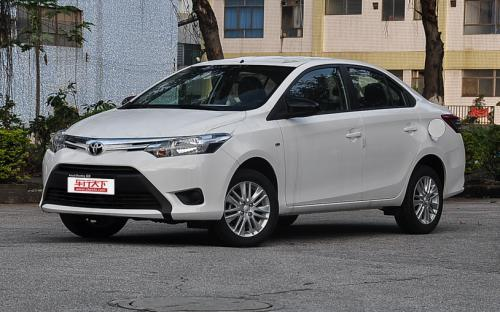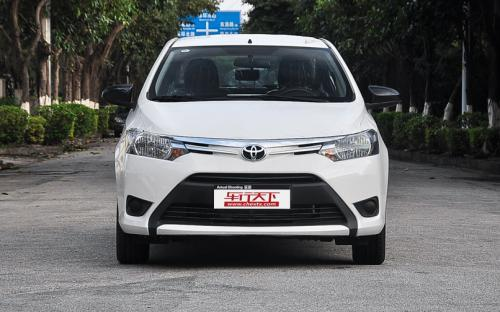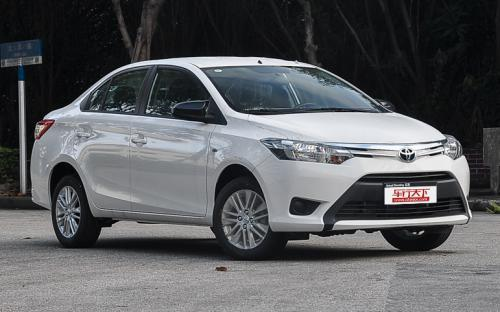### 一汽丰田 威驰0 种颜色可选2016款最低售价：8.18 万元起

4410(mm)1700(mm)1490(mm)##### 配置亮点：
• 胎压监测装置

• ISOFIX儿童座椅接口

• 车身稳定控制(ESC/ESP/DSC等)

• 电动天窗

• 定速巡航

• 后倒车雷达

• 真皮座椅

• GPS导航系统

• 氙气大灯

• 后视镜加热

• 提交
2016款 1.5L 手动智臻星耀版 (98张)
• 2016款 1.5L 手动智臻星耀版 (98张)
• 2016款 1.5L 自动智臻星耀版 (90张)
• 一汽丰田 威驰 绕车实拍• 一汽丰田 威驰 在售车型

排量 车型 厂商指导价 本地最低报价 购车工具
1.5L
1.5L 手动智臻星耀版 停产在售 5挡手动
8.18万
1.5L 自动智臻星耀版 停产在售 4挡自动
8.98万

一汽丰田 威驰 经销商

查看更多 >>

### 一汽丰田 威驰 动力加速

威驰 0-100公里加速时间分布在 0.0-秒 属于 超跑级

动力级别 加速时间 车型

一汽丰田 威驰 视频

一汽丰田 威驰 新闻资讯

# 动力更平顺 一汽丰田新威驰售6.98万起

国产新车 超过10534次关注

2016年9月28日，一汽丰田新款威驰在国内正式上市。新车搭载1.3L和1.5L自然吸气式发动机，升级了自动变速箱。并且对外观、内饰进行了大幅优化。根据不同配置，新车共...

# 售8.18万起 丰田威驰增1.5L智臻星耀版

上市新车 超过9052次关注

一汽丰田正式推出了威驰1.5L智臻星耀版，新车在外观设计方面进行了一些改进，提供手动或自动挡车型选择，售价分别为8.18万和8.98万元。

# 造型运动 丰田威驰TRD Sportivo版官图

进口新车 超过8787次关注

日前，丰田汽车发布了威驰TRDSportivo版车型的官方图片，新车基于海外版威驰打造并装配了TRD车身套件，据悉，这款车将主要面向泰国市场发售。

# 涉及5361辆 一汽丰田召回部分威驰车型

碰撞召回 超过4622次关注

日前，天津一汽丰田汽车有限公司根据《缺陷汽车产品召回管理条例》的要求，向国家质检总局备案了召回计划，决定自2015年1月3日起，召回部分威驰汽车。

# 丰田威驰星光版10月24日上市 9.48万起

国产新车 超过6901次关注

日前，我们获得消息，一汽丰田将会在10月24日正式推出三款威驰星光版车型，这三款车型的售价为9.48-11.08万。

# 售价或低于8万 丰田新威驰跨界版即将到来

车系文章 超过3562次关注

面对销量迅速增长的亚太地区新兴市场，各大汽车企业选择推出低价位车型来扩大市场份额，其中大众、福特是其中的先行者，而将中国视为最重要市场的丰田汽车也有望在...

# 全新威驰1.5L手动智臻版登场

车系文章 超过2951次关注

近日，编辑获悉一汽丰田为全新威驰增配了一款1.5L手动智臻版车型的消息，新车售价为7.98万元。新车型的推出，不仅丰富了威驰的产品线，相对更低的价格也能满足更多...

# 丰田威驰曝光旅行版 流线十足

车系文章 超过2534次关注

一汽丰田新一代威驰在创造了月万辆销售状态之后，外媒绘制出全新威驰旅行版车型的效果图，再一次将威驰推到风口浪尖。不过，目前还没有关于这款新车量产的任何消息。

# 丰田新威驰2014年1-4月销量盘点

车系文章 超过4899次关注

一汽丰田新威驰2014年1月-4月销量：44664辆

# 华丽升级 实拍2014款新威驰

车系文章 超过5428次关注

老款威驰凭着时尚的外观和出色的经济燃油性一直在市场中有着良好的口碑，可由于车型较旧，目前在市场上的销售比较低迷。在近日一汽丰田又发布了2014款全新威驰轿车...

# 丰田新锐 试驾评测新款一汽丰田威驰

车系文章 超过3003次关注

11年前，一汽丰田威驰在中国上市的时候，我才刚刚13岁；那时候的我并不知道它是丰田导入中国市场的第一款国产车，更不知道它是丰田第一款为中国市场打造的车型。

猜你喜欢

﻿
• 快速找车
• 选择品牌
• 选择品牌
• A  奥迪
• A  阿斯顿·马丁
• A  阿尔法·罗密欧
• B  宝沃
• B  布加迪
• B  巴博斯
• B  保时捷
• B  宾利
• B  奔驰
• B  宝马
• B  本田
• B  别克
• B  标致
• B  比亚迪
• B  宝骏
• B  北汽制造
• B  北汽新能源
• B  北汽幻速
• B  北汽威旺
• B  北京汽车
• B  奔腾
• B  北汽绅宝
• C  长安
• C  长安商用
• C  长城
• C  昌河
• D  大众
• D  道奇
• D  DS
• D  东南
• D  东风风神
• D  东风风行
• D  东风小康
• D  东风风度
• D  东风
• F  福特
• F  丰田
• F  菲亚特
• F  法拉利
• F  福田
• F  福迪
• F  福汽启腾
• G  观致
• G  广汽传祺
• G  广汽吉奥
• G  GMC
• H  红旗
• H  汉腾汽车
• H  哈弗
• H  哈飞
• H  海格
• H  海马
• H  华颂
• H  黄海
• H  华泰
• H  恒天
• J  吉利汽车
• J  捷豹
• J  Jeep
• J  江淮
• J  江铃
• J  金杯
• J  九龙
• J  金旅
• K  凯翼
• K  凯迪拉克
• K  克莱斯勒
• K  科尼塞克
• K  卡威
• K  开瑞
• L  路虎
• L  林肯
• L  劳斯莱斯
• L  兰博基尼
• L  雷克萨斯
• L  铃木
• L  雷诺
• L  理念
• L  力帆
• L  莲花汽车
• L  猎豹
• L  路特斯
• L  陆风
• M  马自达
• M  MG
• M  MINI
• M  玛莎拉蒂
• M  摩根
• M  迈凯轮
• N  纳智捷
• O  欧宝
• O  讴歌
• O  欧朗
• Q  奇瑞
• Q  起亚
• Q  启辰
• R  日产
• R  荣威
• R  瑞麒
• S  三菱
• S  斯威汽车
• S  萨博
• S  smart
• S  斯柯达
• S  斯巴鲁
• S  思铭
• S  双龙
• S  上汽大通
• S  双环
• T  特斯拉
• T  腾势
• W  沃尔沃
• W  五菱汽车
• W  五十铃
• W  威兹曼
• W  威麟
• X  现代
• X  雪佛兰
• X  雪铁龙
• X  西雅特
• Y  一汽
• Y  英菲尼迪
• Y  英致
• Y  依维柯
• Y  野马汽车
• Y  永源
• Z  众泰
• Z  中华
• Z  中兴
• Z  知豆
• 选择车系
• 选择车系
• 车型对比
• 选择品牌
• 选择品牌
• A  奥迪
• A  阿斯顿·马丁
• A  阿尔法·罗密欧
• B  宝沃
• B  布加迪
• B  巴博斯
• B  保时捷
• B  宾利
• B  奔驰
• B  宝马
• B  本田
• B  别克
• B  标致
• B  比亚迪
• B  宝骏
• B  北汽制造
• B  北汽新能源
• B  北汽幻速
• B  北汽威旺
• B  北京汽车
• B  奔腾
• B  北汽绅宝
• C  长安
• C  长安商用
• C  长城
• C  昌河
• D  大众
• D  道奇
• D  DS
• D  东南
• D  东风风神
• D  东风风行
• D  东风小康
• D  东风风度
• D  东风
• F  福特
• F  丰田
• F  菲亚特
• F  法拉利
• F  福田
• F  福迪
• F  福汽启腾
• G  观致
• G  广汽传祺
• G  广汽吉奥
• G  GMC
• H  红旗
• H  汉腾汽车
• H  哈弗
• H  哈飞
• H  海格
• H  海马
• H  华颂
• H  黄海
• H  华泰
• H  恒天
• J  吉利汽车
• J  捷豹
• J  Jeep
• J  江淮
• J  江铃
• J  金杯
• J  九龙
• J  金旅
• K  凯翼
• K  凯迪拉克
• K  克莱斯勒
• K  科尼塞克
• K  卡威
• K  开瑞
• L  路虎
• L  林肯
• L  劳斯莱斯
• L  兰博基尼
• L  雷克萨斯
• L  铃木
• L  雷诺
• L  理念
• L  力帆
• L  莲花汽车
• L  猎豹
• L  路特斯
• L  陆风
• M  马自达
• M  MG
• M  MINI
• M  玛莎拉蒂
• M  摩根
• M  迈凯轮
• N  纳智捷
• O  欧宝
• O  讴歌
• O  欧朗
• Q  奇瑞
• Q  起亚
• Q  启辰
• R  日产
• R  荣威
• R  瑞麒
• S  三菱
• S  斯威汽车
• S  萨博
• S  smart
• S  斯柯达
• S  斯巴鲁
• S  思铭
• S  双龙
• S  上汽大通
• S  双环
• T  特斯拉
• T  腾势
• W  沃尔沃
• W  五菱汽车
• W  五十铃
• W  威兹曼
• W  威麟
• X  现代
• X  雪佛兰
• X  雪铁龙
• X  西雅特
• Y  一汽
• Y  英菲尼迪
• Y  英致
• Y  依维柯
• Y  野马汽车
• Y  永源
• Z  众泰
• Z  中华
• Z  中兴
• Z  知豆
• 选择车系
• 选择车系
• 选择车型
• 选择车型
• 意见反馈